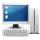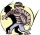# Investment 2

Jack invested \$5000 in a 5-month term deposit at 4.7% p. A. . At the end of the 5-months, jack reinvested the maturity value from the first deposit into an 11-month term deposit at 7.3% p. A. What is the maturity value at the end of the second term deposit?

Result

c =  5436.644 USD

#### Solution:Leave us a comment of example and its solution (i.e. if it is still somewhat unclear...):

Showing 0 comments:Be the first to comment!## Next similar examples:

1. Investment1000\$ is invested at 10% compound interest. What factor is the capital multiplied by each year? How much will be there after n=12 years?
2. Saving 9An amount if \$ 2000 is invested at an interest of 5% per month. if \$ 200 is added at the beginning of each successive month but no withdrawals. Give an expression for the value accumulated after n months. After how many months will the amount have accumula
3. Account operationsMy savings of php 90,000 in a bank earns 6% interest in a year. If i will deposit additional php 10,000 at the end of 6 months, how much money will be left if i withdraw php 25,000 after a year?
4. BankPaul put 10000 in the bank for 6 years. Calculate how much you will have in the bank if he not pick earned interest or change deposit conditions. The annual interest rate is 3.5%, and the tax on interest is 10%.
5. DepositIf you deposit 719 euros the beginning of each year, how much money we have at 1.3% (compound) interest after 9 years?
6. Six termsFind the first six terms of the sequence a1 = -3, an = 2 * an-1
7. Annual pensionCalculate the amount of money generating an annual pension of EUR 1000, payable at the end of the year and for a period of 10 years, shall be inserted into the bank to account with an annual interest rate of 2%
8. ComputerThe computer was purchased 10000,-. Each year, the price of a computer depreciates always the same percentage of the previous year. After four years, the value of the computer is reduced to 1300,- How many percent was depreciated price of the computer each
9. Five membersWrite first 5 members geometric sequence and determine whether it is increasing or decreasing: a1 = 3 q = -2
10. PopulationWhat is the population of the city with 3% annual growth, if in 10 years the city will have 60,000 residents?
11. GP - 8 itemsDetermine the first eight members of a geometric progression if a9=512, q=2
12. Tenth memberCalculate the tenth member of geometric sequence when given: a1=1/2 and q=2
13. Geometric sequence 4It is given geometric sequence a3 = 7 and a12 = 3. Calculate s23 (= sum of the first 23 members of the sequence).
14. Theorem proveWe want to prove the sentence: If the natural number n is divisible by six, then n is divisible by three. From what assumption we started?
15. Geometric progression 2There is geometric sequence with a1=5.7 and quotient q=-2.5. Calculate a17.
16. Radioactive materialA radioactive material loses 10% of its mass each year. What proportion will be left there after n=6 years?
17. The crimeThe crime rate of a certain city is increasing by exactly 7% each year. If there were 600 crimes in the year 1990 and the crime rate remains constant each year, determine the approximate number of crimes in the year 2025.